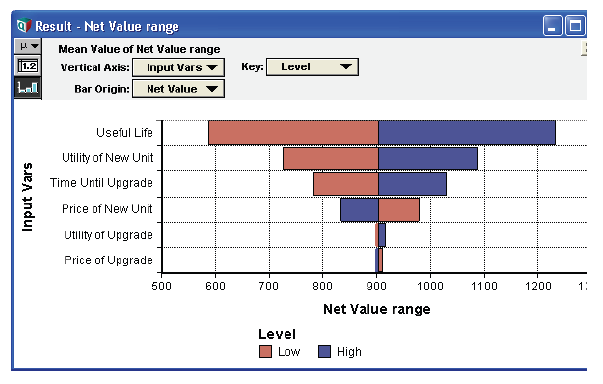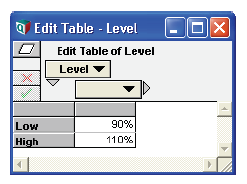(diff) ← Older revision | Latest revision (diff) | Newer revision → (diff)

Release: 4.6  •  5.0  •  5.1  •  5.2  •  5.3  •  5.4  •  6.0  •  6.1  •  6.2  •  6.3  •  6.4

A tornado diagram is a common tool used to depict the sensitivity of a result to changes in selected variables. It shows the effect on the output of varying each input variable at a time, keeping all the other input variables at their initial (nominal) values. Typically, you choose a “low” and a “high” value for each input. The result is then displayed as a special type of bar graph, with bars for each input variable displaying the variation from the nominal value. It is standard practice to plot the bars horizontally, sorted so that the widest bar is placed at the top. When drawn in this fashion, the diagram takes on the appearance of a tornado, hence its name. The figure below shows a typical tornado diagram.1. Select the result or output variable to perform the analysis on.
2. Select the input variables that might affect the output.
3. Decide what the low and high values are to be for each input variable.

Note: The input variables do not need to be chance variables. In fact, tornado analysis is often applied to models with no chance variables.

There are several options for selecting low and high values, including:

• Selecting the same absolute low and high levels for every input. This usually only makes sense if inputs are very homogeneous with identical nominal values.
• Selecting absolute low and high values separately for each input variable.
• Varying all inputs by the same relative amount, e.g., low = 90% of nominal, high = 110% of nominal.
• Varying all inputs between two given fractiles (aka percentiles, quartiles). This only makes sense if your inputs are uncertain variables. Example: Low = 10% fractile, High = 90% fractile, nominal = 50% fractile.

For this example, assume we vary all inputs by the same amount.

1. Create an index variable containing a list of input variable identifiers. Suppose this is called Vars.
2. Create a variable, Level, and define it as a self-indexed table. (To do this, select Table from the expr menu, and select self as an index.) From the edit table, set the self-index labels to read low and high. Set the value corresponding to low to 90%, and set the value corresponding to high to 110%.3. Create a node, Tornado_Analysis. Assume that the output variable is Net_value. Define Tornado_Analysis as:
WhatIfAll(X, Vars, Level*Vars)

It is possible in Analytica to use array abstraction to produce a set of tornado diagrams, with each tornado itself indexed by an additional dimension. Additional dimensions are already included if your output variable is itself an array result, in which case you have a tornado diagram for each element in the output value’s array value.

The WhatIfAll function typically provides the easiest method for implementing a tornado analysis in Analytica. Note that the third parameter to WhatIfAll controls the method by which inputs are varied for the analysis. For example:

• For the case where you select the same absolute low and high levels for every input, Level would be set to the absolute low and high values, and the third parameter to WhatIfAll would be simply Level.
• For the case where you select absolute low and high values separately for each input variable, you would index Level by Vars, fill in Level’s table appropriately, then set the third parameter to be just Level.
• And for the case where you vary all inputs between two given fractiles, you would set Level to the desired fractiles, and use the expression GetFract(Net_value, Level) as the third parameter.

It’s customary to graph a tornado with the names of the input variables are listed down the vertical axis, and the bars displaying the effect on the output horizontally:

1. Select Show Result for the Tornado_Analysis variable. Press the Graph button if necessary.
2. Pivot the index order (if necessary) so that Vars is on the X-axis and L is the Key.
3. Select Graph Setup and the Chart Type tab.
4. Set the Line Style to the filled bar setting and check the Variable origin checkbox. This will also set Bar Overlap = 100% and Swap horizontal and vertical for you.
5. Set the Sort by data spread checkbox.
6. Click Apply.
7. Next, we want to compare to the baseline value of Net_Value. Click the XY button to open the XY Comparison Sources dialog, check Use another variable, press Add..., and in the Object Finder select the variable Net_Value. Press OK twice.
8. In the Bar Origin pulldown, select Net_value.